﻿ 双馈风电机组接入对电力系统暂态功角稳定性的影响

# 双馈风电机组接入对电力系统暂态功角稳定性的影响Influence of DFIG on Transient Power Angle Stability of Power System

Abstract: Due to the randomness, intermittence and non controllability of wind power station, the access of large-scale wind power will change the original power flow distribution and the inertia of the whole system. These will have an impact on the transient stability of the power system after the wind power is connected to the grid. This paper analyzes the power characteristics of a doubly-fed wind turbine after it is connected to a power system. This paper also studies the transient power angle stability of doubly-fed wind turbine connected to single ended power transmission system, and analyzes the impact on the stability of the system transient power angle.

1. 引言

2. 双馈风电机组的基本结构与数学模型

2.1. 基本结构

2.2. 数学模型

1) 电机定、转子绕组三相对称。

2) 双馈电机磁路关于直轴d和交轴q对称，且q轴超前d轴90˚。

3) 绕组中的电流在空间和气隙中产生的磁动势沿电枢内侧正弦分布。

4) 双馈电机定、转子绕组正方向采用电动机惯例，流入绕组方向为电流正方向，正的电流产生正的磁链。

5) 忽略谐波、忽略磁滞饱和铁芯损耗。

6) 忽略温度和频率变化对电机参数的影响。

dq同步旋转坐标系下的数学模型如下：

$\left\{\begin{array}{l}{u}_{ds}={R}_{s}{i}_{ds}+P{\psi }_{ds}-{\omega }_{s}{\psi }_{qs}\\ {u}_{qs}={R}_{s}{i}_{qs}+P{\psi }_{qs}+{\omega }_{s}{\psi }_{ds}\end{array}$ (1)

$\left\{\begin{array}{l}{u}_{dr}={R}_{r}{i}_{dr}+P{\psi }_{dr}-\left({\omega }_{s}-{\omega }_{r}\right){\psi }_{qr}\\ {u}_{qr}={R}_{r}{i}_{qr}+P{\psi }_{qr}+\left({\omega }_{s}-{\omega }_{r}\right){\psi }_{dr}\end{array}$ (2)

$\left\{\begin{array}{l}{\psi }_{ds}={L}_{s}{i}_{ds}+{L}_{m}{i}_{dr}\\ {\psi }_{qs}={L}_{s}{i}_{qs}+{L}_{m}{i}_{qr}\end{array}$ (3)

$\left\{\begin{array}{l}{\psi }_{dr}={L}_{s}{i}_{ds}+{L}_{r}{i}_{dr}\\ {\psi }_{qr}={L}_{s}{i}_{qs}+{L}_{r}{i}_{qr}\end{array}$ (4)

${T}_{e}=p\left({\psi }_{ds}{i}_{qs}-{\psi }_{qs}{i}_{ds}\right)$ (5)

$\left\{\begin{array}{l}{P}_{s}={u}_{ds}{i}_{ds}+{u}_{qs}{i}_{qs}\\ {Q}_{s}={u}_{qs}{i}_{ds}-{u}_{ds}{i}_{qs}\end{array}$ (6)

3. 双馈风电机组对电力系统暂态功角稳定性的影响

3.1. 双馈风电机组的外特性

1) 故障发生前以及故障恢复后的后期双馈风电机组的等效外特性故障发生前以及故障恢复后期，双馈风电机组运行在恒功率因数运行方式下，其等效外特性为一恒定的负电阻 $-r$

2) 故障期间以及故障恢复后的前期双馈风电机组的等效外特性。

${\stackrel{˙}{\psi }}_{s}=\frac{h{\stackrel{˙}{U}}_{s}}{{\omega }_{s}}+\left(1-h\right)\frac{{\stackrel{˙}{U}}_{s}}{{\omega }_{s}}{\text{e}}^{-\left({R}_{s}/{L}_{s}+j{\omega }_{s}\right)t}$ (7)

${P}_{g}=-\left(1-s\right)\frac{{E}^{\prime }{U}_{s}}{{x}^{\prime }}\mathrm{sin}{\delta }_{g}$ (8)

$\left\{\begin{array}{l}{\stackrel{˙}{\psi }}_{s}=\frac{h{\stackrel{˙}{U}}_{s}}{{\omega }_{s}}\\ {\stackrel{˙}{\psi }}_{r}=\frac{{L}_{m}}{{L}_{s}}\cdot \frac{h{\stackrel{˙}{U}}_{s}}{{\omega }_{s}}\end{array}$ (9)

$r={u}_{s}^{2}/{p}_{g}=-\frac{{u}_{s}^{2}}{\left(1-s\right)\frac{{e}^{\prime }{u}_{s}}{{x}^{\prime }}\mathrm{sin}{\delta }_{g}}=-\frac{{\psi }_{s}{x}^{\prime }}{\left(1-s\right)\frac{{L}_{m}}{{L}_{r}}{\psi }_{r}\mathrm{sin}{\delta }_{g}}\approx -\frac{{x}^{\prime }}{\mathrm{sin}{\delta }_{g}}$ (10)

$x={u}_{s}^{2}/{q}_{g}=-\frac{{u}_{s}^{2}}{\frac{{\left({e}^{\prime }\right)}^{2}}{{x}^{\prime }}-\frac{{e}^{\prime }{u}_{s}}{{x}^{\prime }}\mathrm{cos}{\delta }_{g}}\approx -\frac{{x}^{\prime }}{1-\mathrm{cos}{\delta }_{g}}$ (11)

$\left\{\begin{array}{l}r=-\frac{{x}^{\prime }}{\mathrm{sin}{\delta }_{g}}\\ x=-\frac{{x}^{\prime }}{1-\mathrm{cos}{\delta }_{g}}\end{array}$ (12)

3.2. 双馈风电机组接入对同步发电机组功率特性的影响

1) 故障发生前以及故障恢复后期双馈风电机组接入后同步机组的功率特性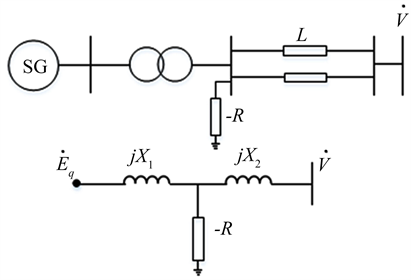Figure 1. Schematic diagram of single end power transmission system

${P}_{Eq}=\frac{{E}_{q}^{2}}{|{Z}_{11}|}\mathrm{sin}{\alpha }_{11}+\frac{{E}_{q}V}{|{Z}_{12}|}\mathrm{sin}\left(\delta -{\alpha }_{12}\right)$ (13)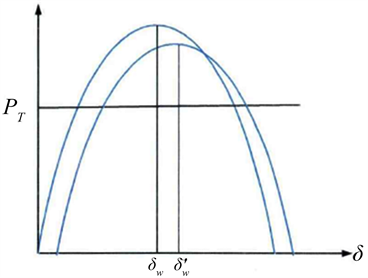Figure 2. Schematic diagram of power characteristic curve of synchronous unit after DFIG is connected

2) 故障期间以及故障恢复初期双馈风电机组接入后同步机组的的功率特性单端送电系统示意图如图3所示。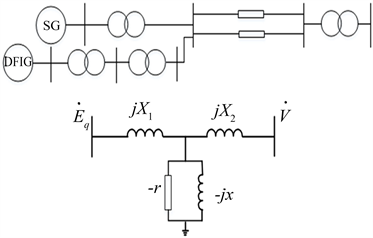Figure 3. Including DFIG single terminal power transmission system

$z={X}_{1}{X}_{2}\left(\frac{1}{r}-j\frac{1}{x}\right)=\frac{{X}_{1}{X}_{2}}{x}\left(\mathrm{sin}{\delta }_{g}-j\left(1-\mathrm{cos}{\delta }_{g}\right)\right)$ (14)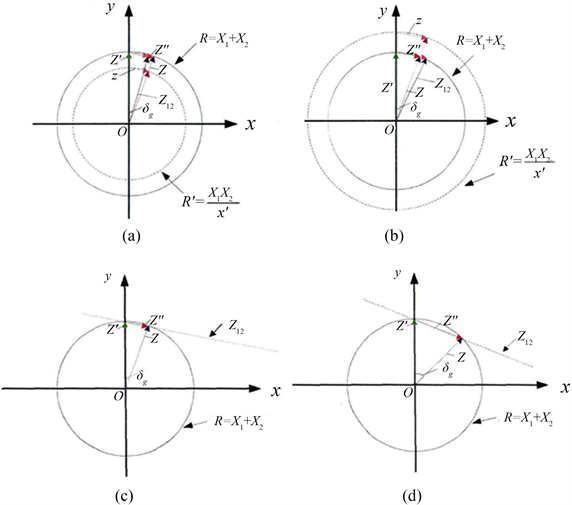Figure 4. Transfer impedance vector diagram

$Z={Z}^{\prime }+{Z}^{″}$ (15)

$\left\{\begin{array}{l}Z={Z}^{\prime }{\text{e}}^{-j{\delta }_{g}}\\ {Z}^{″}=\left({X}_{1}+{X}_{2}\right)\mathrm{sin}{\delta }_{g}+j\left({X}_{1}+{X}_{2}\right)\left(1-\mathrm{cos}{\delta }_{g}\right)\end{array}$ (16)

3.3. 并网DFIG与系统暂态功角稳定模式的交互影响

1) 从并网DFIG的角度来看，并网DFIG的功率输出特性和自身的暂态运行失稳机制都是主要取决于其机端电压的跌落程度和变流器控制系统的控制策略及其暂态稳定性，而与系统内同步发电机组的转速和转子角的暂态行为没有直接关联，因此实现了强机电解耦的并网DFIG在系统暂态过程中不像传统同步发电机组一样直接受到系统暂态功角稳定模式的制约。

2) 从电网的角度来看，并网DFIG通过交流励磁实现了转子机械量与发电机定子电气量的解耦，使得并网DFIG不能像同步发电机一样为同步系统提供直接的惯性支持，因此并网的DFIG的转速信息和等效功角既无法直接反映DFIG的稳定状态，而对于系统内的同步发电机组构造互同步稳定机制也没有直接的物理意义。

3.4. 双馈风电机组接入下的单机系统暂态功角稳定分析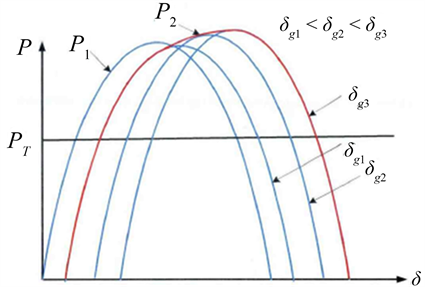Figure 5. Schematic diagram of power characteristics of DFIG connected to the back-end system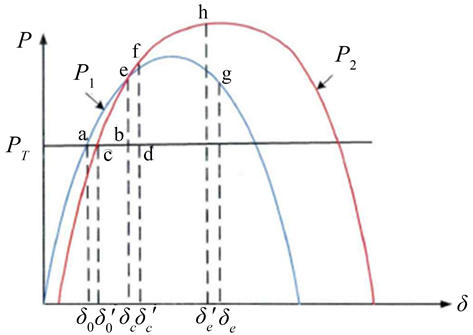Figure 6. Equal area rule of sending end system during and before fault clearing

4. 结论

DFIG接入单端送电系统影响了电力系统暂态功角稳定性，并网DFIG通过其功率输出特性影响系统内同步发电机的暂态功率响应，进而影响系统内同步发电机的转子运动情况和功角稳定性，是其参与影响系统暂态功角稳定的基本方式。

 郝正航. 双馈风电机组的暂态行为及其对电力系统稳定性影响[D]: [博士学位论文]. 天津: 天津大学, 2011.

 李帅兵. 前端调速式风电机组并网特性研究[D]: [博士学位论文]. 兰州: 兰州交通大学, 2014.

 王阔. 风火打捆外送系统暂态稳定性及控制策略研究[D]: [博士学位论文]. 兰州: 兰州理工大学, 2016.

 马敏. 风电接入对电网暂态稳定性及调峰能力的影响研究[D]: [博士学位论文]. 北京: 华北电力大学, 2011.

 邓丽. 风电并网对电力系统安全稳定的影响[D]: [博士学位论文]. 济南: 山东大学, 2013.

 刘斯伟. 并网双馈风电机组对电力系统暂态稳定性的影响机理研究[D]: [博士学位论文]. 北京: 华北电力大学, 2016.

 张竹生. 微分半动力系统的不变集[D]: [博士学位论文]. 北京: 北京大学数学系, 1983.

 林旻威. 大规模风电接入对电力系统暂态稳定性影响研究综述[J]. 电气技术, 2017, 12(1): 3-8.

 杨帆. 大规模风电机组连锁脱网仿真分析及对策[J]. 电力科学与工程, 2013, 35(5): 2-7.

Top